GeeksforGeeks App
Open AppBrowser
Continue

# Visualization and Prediction of Crop Production data using Python

Prerequisite: Data Visualization in Python

Visualization is seeing the data along various dimensions. In python, we can visualize the data using various plots available in different modules.

In this article, we are going to visualize and predict the crop production data for different years using various illustrations and python libraries.

### Dataset

The Dataset contains different crops and their production from the year 2013 – 2020.

### Requirements

There are a lot of python libraries which could be used to build visualization like matplotlib, vispy, bokeh, seaborn, pygal, folium, plotly, cufflinks, and networkx. Of the many, matplotlib and seaborn seems to be very widely used for basic to intermediate level of visualizations.

However, two of the above are widely used for visualization i.e.

• Matplotlib: It is an amazing visualization library in Python for 2D plots of arrays, It is a multi-platform data visualization library built on NumPy arrays and designed to work with the broader SciPy stack. Use the below command to install this library:
`pip install matplotlib`
• Seaborn: This library sits on top of matplotlib. In a sense, it has some flavors of matplotlib while from the visualization point, it is much better than matplotlib and has added features as well. Use the below command to install this library:
`pip install seaborn`

### Step-by-step Approach

• Import required modules
• Display the data and constraints of the loaded dataset.
• Use different methods to visualize various illustrations from the data.

### Visualizations

Below are some programs which indicates the data and illustrates various visualizations of that data:

Example 1:

## Python3

 `# importing pandas module``import` `pandas as pd` `# load the dataset``data ``=` `pd.read_csv(``'crop.csv'``)` `# display top 5 values``data.head()`

Output: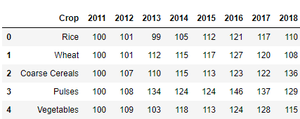These are the top 5 rows of the dataset used.

Example 2:

## Python3

 `# data description``data.info()`

Output: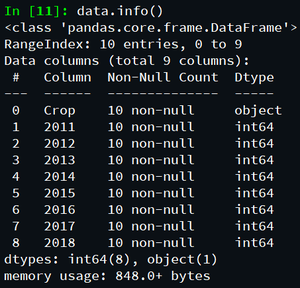These are the data constraints of the dataset.

Example 3:

## Python3

 `# 2011 crop data in histogram analysis``data[``'2011'``].hist()`

Output: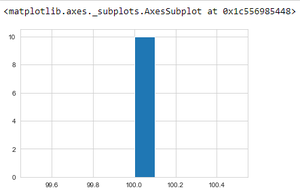The above program depicts the crop production data in the year 2011 using histogram.

Example 4:

## Python3

 `# 2012 crop data in histogram analysis``data[``'2012'``].hist()`

Output: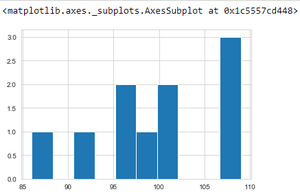The above program depicts the crop production data in the year 2012 using histogram.

Example 4:

## Python3

 `# 2013 crop data in histogram analysis``data[``'2013'``].hist()`

Output: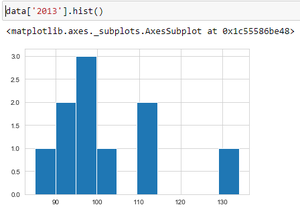The above program depicts the crop production data in the year 2013 using histogram.

Example 5:

## Python3

 `# display all year data``data.hist()`

Output: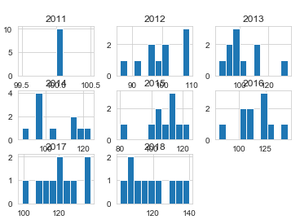The above program depicts the crop production data of all the available time periods(year) using multiple histograms.

Example 6:

## Python3

 `# import seaborn module``import` `seaborn as sns` `# setting style``sns.set_style(``"whitegrid"``)` `# plotting data using boxplot for 2013 - 2014``sns.boxplot(x``=``'2013'``, y``=``'2014'``, data``=``data)`

Output: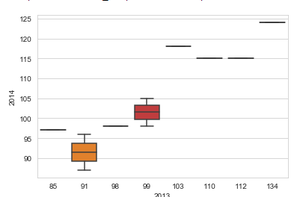Comparing crop productions in the year 2013 and 2014 using box plot.

Example 7:

## Python3

 `# scatter plot 2013 data vs 2014 data``plt.scatter(data[``'2013'``],data[``'2014'``])` `plt.show()`

Output: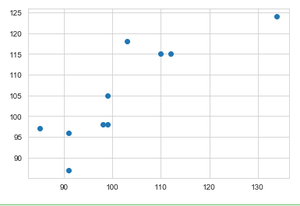Comparing crop production in the year 2013 and 2014 using scatter plot.

Example 8:

## Python3

 `# line plot 2013 data vs 2014 data``plt.plot(data[``'2013'``],data[``'2014'``])` `plt.show()`

Output: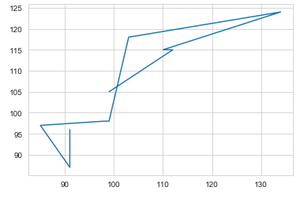Comparing crop productions in the year 2013 and 2014 using line plot.

Example 9:

## Python3

 `# import required modules``import` `matplotlib.pyplot as plt``from` `scipy ``import` `stats`  `# assign data``x ``=` `data[``'2017'``]``y ``=` `data[``'2018'``]`  `# linear regression 2017 data vs 2018 data``slope, intercept, r, p, std_err ``=` `stats.linregress(x, y)`  `# function to return slope``def` `myfunc(x):``    ``return` `slope ``*` `x ``+` `intercept`  `mymodel ``=` `list``(``map``(myfunc, x))` `# scatter``plt.scatter(x, y)` `# plotting the data``plt.plot(x, mymodel)` `# display the figure``plt.show()`

Output: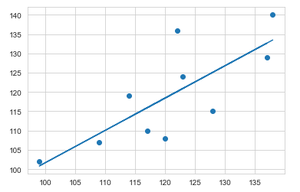Applying linear regression to visualize and compare predicted crop production data between the year 2017 and 2018.

Example 10:

## Python3

 `# import required modules``import` `matplotlib.pyplot as plt``from` `scipy ``import` `stats`  `# assign data``x ``=` `data[``'2016'``]``y ``=` `data[``'2017'``]`  `# linear regression 2017 data vs 2018 data``slope, intercept, r, p, std_err ``=` `stats.linregress(x, y)`  `# function to return slope``def` `myfunc(x):``    ``return` `slope ``*` `x ``+` `intercept`  `mymodel ``=` `list``(``map``(myfunc, x))` `# scatter``plt.scatter(x, y)` `# plotting the data``plt.plot(x, mymodel)` `# display the figure``plt.show()`

Output: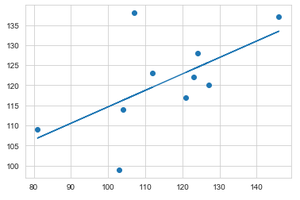Applying linear regression to visualize and compare predicted crop production data between the year 2016 and 2017.

### Demo Video

This video shows how to depict the above data visualization and predict data, using Jupyter Notebook from scratch.

In this way various data visualizations and predictions can be computed.

My Personal Notes arrow_drop_up